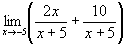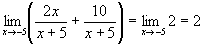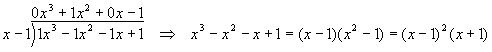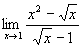Limits - Techniques 1 - Finite fixed point (x0), finite limit (y0)

Here we are less interested in the definition of the limit than in how to calculate them. We proceed by examples, using the following terminologyf(x) is the function, with all possible x values the domain of the function, all possible y values the range of the function
x0 is our fixed point, can be finite or infinite, but must be in the domain of the function
y0 is the limit, can be finite, infinite, or non-existent, if it exists, it must be in the range of the function

Find the limitSolution

Clearly we'll have problems just substituting x = 1, since that will make the denominator zero, and the entire expression undefined.  Instead, we'll do some algebra first to see if we can trim off any fat.  Since (see algebra formulae)We can writeAnd soFind the limitSolution

Again, straight substitution won't work. In fact, regardless of how we play with this limit, we will still have a factor in the denominator which is zero, while the numerator is not. This limit does not exist. We cannot even assign it either +/- infinity, since the answer will be different depending on whether x approaches 4 from below (negative infinity) or above (positive infinity).

Find the limitSolution

Apply the same algebra technique as the first example above, twice:So,Find the limitSolution

A little algebra reveals that this limit is also not what it seemsAnd soSince 2 is not a function of x, it doesn't matter what the fixed point is, the answer is still 2!

Find the limitFor the three cases: m > n, m = n, m < n.

Solution

The m = n case is trivialIn the case where m < n or m > n, from the algebra formulaeSo,Notice that this agrees with the trivial result when m = n.

Find the limitSolution

Multiply by 1 in the form of the numerator with a "+" sign substituted for a "-" sign:Therefore,Please note in the above examples that, once the limit has been taken, the limit symbol is removed and the fixed point is substituted for x. Prior to that, the limit symbol is needed. When we are doing pure algebra, we leave off the limit symbol to avoid cluttering the math.

Find the limitSolution

Inspection of the denominator shows it becomes zero at x = 1, which means (x - 1) is a factor of the polynomial in the denominator. Performing a division (you can do the subtraction under the divisor if you like - you will need to be able to factor cubic polynomials like this),Since x = 1 is an interesting point for the denominator, we can try it with the numeratorThe quadratic term on the right is irreducible, sinceSo, out limit becomesThe numerator becomes finite while the denominator goes to zero, there is no more algebra which can reduce the expression, so this limit does not exist.

Find the limitSolution

Here it helps to do a substitutionso that we can now write the limit expression asThis technique will prove very useful for calculus problems later.

Recommended Books

The classic calculus problem book - very light on theory, plenty of problems with full solutions, more problems with answers

A simplified and updated version of the classic Schaum's Outline. Not as complete as the previous book, but enough for most students